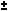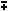# find the complete solution for it :-tanx + tany = 4,tan3x + tan3y =2

10 years ago

Dear Suraj,

tan(xy) = (tan xtan y) / (1tan x tan y)

sin(2x) = 2 sin x cos x

cos(2x) = cos^2(x) - sin^2(x) = 2 cos^2(x) - 1 = 1 - 2 sin^2(x)

tan(2x) = 2 tan(x) / (1 - tan^2(x))

sin^2(x) = 1/2 - 1/2 cos(2x)

cos^2(x) = 1/2 + 1/2 cos(2x)

sin x - sin y = 2 sin( (x - y)/2 ) cos( (x + y)/2 )

cos x - cos y = -2 sin( (x - y)/2 ) sin( (x + y)/2 )

Cracking IIT just got more exciting,It s not just all about getting assistance from IITians, alongside Target Achievement and Rewards play an important role. ASKIITIANS has it all for you, wherein you get assistance only from IITians for your preparation and win by answering queries in the discussion forums.

So start the brain storming…. become a leader with Elite Expert League ASKIITIANS

Thanks

Aman Bansal